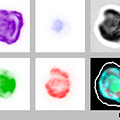# Question regarding 5d CONV

Hi All, I come here to try to look for some experienced advice.

I’m working with a 3D image dataset wrapped into a GIF file.

Each Gif file has 20 slices of 5 different images, representing a 3D object.Each Gif File has 4 different florescent filters and the original image as a 5th filter.

When passing the Gif file into a tensor it’s size is: [ f, s, w, h]

f is the number of filters of the image = 5
s is the number of slices of each filter = 20
w is the width of each filter = 121
h is the height of each filter = 121

Now the idea is to generate a model that could distinguish different classes in the images.

My question is the following, is there any way where I could use the 5 filters as inputs without having to implement a 5D convolution ??

Nowadays I have chosen 3 of those 5 filters and used a C3D model as a POC and looking forward to use a 3D Resnet, but I would love to be able to use all the input information instead of just 3/5.

Any thoughts??

Github of the project: https://github.com/fmcalcagno/TaraPlanktonRecognition

Why would you use a 5D convolution? I would suggest a 3D convolution or a 2D + t solution like a 2d-conv-RNN.

If using a 3D convolution you would use `f` as number of channels, `s` as depth and `h` and `w` as height and width. Adding a batchsize should work out of the box.

If using a 2d+t approach you would have to transpose the `f` and the `s` axis, using the `f` axis as input channels, `h` and `w` as dimensions and the `s` axis as time axis for recurrence.

1 Like

Thanks, I was a bit confused I guess when talking about 3D convolution. I’ll start implementing the 3D convolution with 5 filters and let you know!
Thanks for the support!